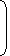# Important Formulas of Pipes and Cistern1. Inlet:A pipe connected with a tank or a cistern or a reservoir, that fills it, is known as an inlet.Outlet:

A pipe connected with a tank or cistern or reservoir, emptying it, is known as an outlet.

2. If a pipe can fill a tank in x hours, then:
 part filled in 1 hour = 1 . x
3. If a pipe can empty a tank in y hours, then:
 part emptied in 1 hour = 1 . y
4. If a pipe can fill a tank in x hours and another pipe can empty the full tank in y hours (where y > x), then on opening both the pipes, then
 the net part filled in 1 hour =1 – 1. x y
5. If a pipe can fill a tank in x hours and another pipe can empty the full tank in y hours (where x > y), then on opening both the pipes, then
 the net part emptied in 1 hour =1 – 1. y x

Correct! Wrong!

Correct! Wrong!

Correct! Wrong!

Correct! Wrong!

Correct! Wrong!

HURRAHHHH!!!!!!!